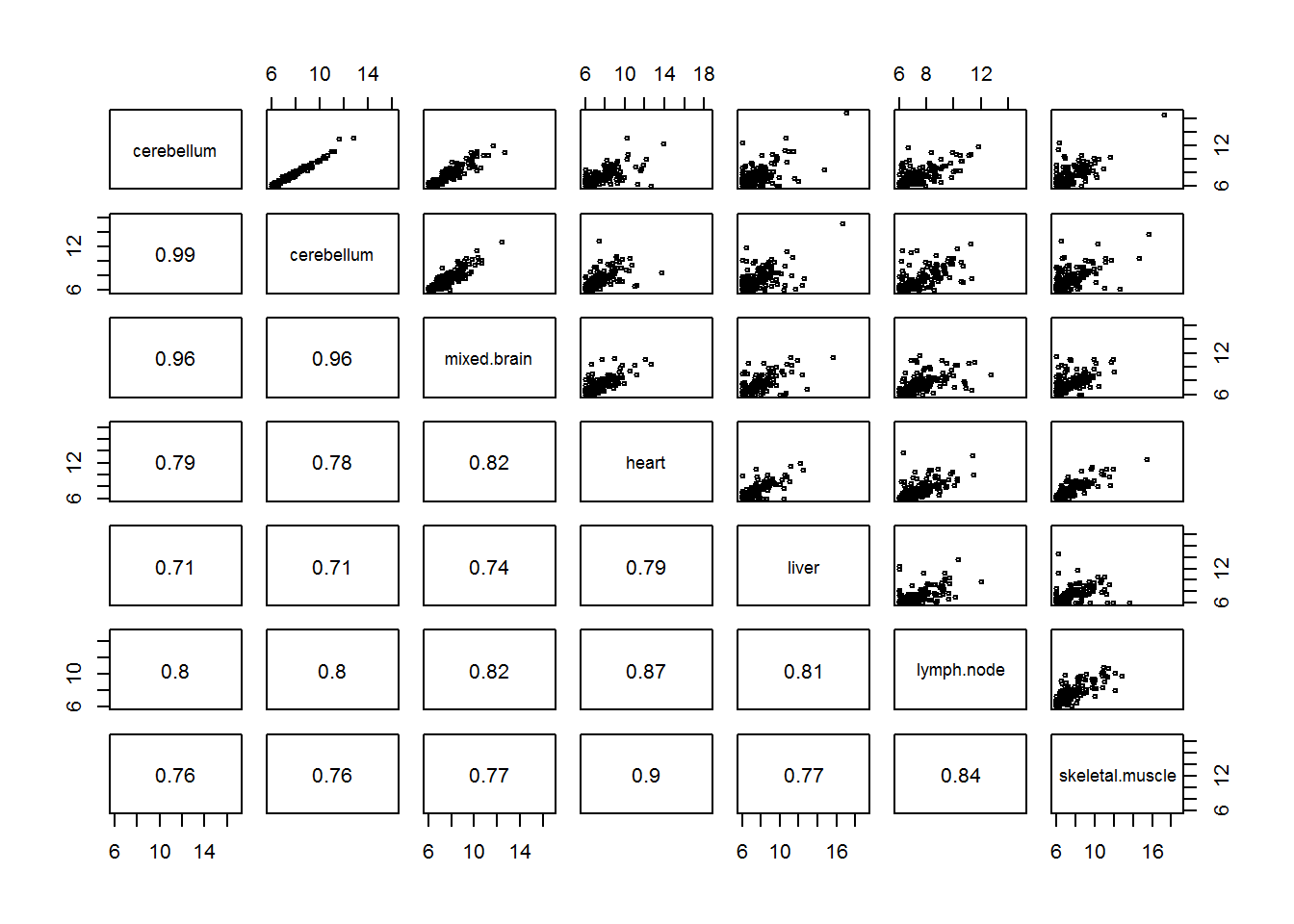### Sample data information

library(airway)
dir <- system.file("extdata", package="airway", mustWork=TRUE) #Specifies data file path in airway package
csv.file <- file.path(dir, "sample_table.csv") #File path for sample info table
bam.files <- file.path(dir, paste0(sample.table$Run, "_subset.bam")) #File paths for each sample .bam data gtf.file <- file.path(dir, "Homo_sapiens.GRCh37.75_subset.gtf") #File path for file containing exon info library(Rsamtools) bam.list <- BamFileList(bam.files) #BamFileList object that stores data in from all bam files library(GenomicFeatures) txdb <- makeTxDbFromGFF(gtf.file, format="gtf") #TxDb object that stores transcript annotation exons.by.gene <- exonsBy(txdb, by="gene") #Creates GRangesList • TxDb objects are RSQLite objects that can use saveDb() and loadDb() • In GRangesList, each element is a table of exons for a single gene • length() will return number of genes in list, elementLengths() will return number of exons for each gene Generating counts with Genomic Alignments package library(GenomicAlignments) #Generate a SummarizedExperiment object containing gene/exon counts se <- summarizeOverlaps(exons.by.gene, bam.list, mode="Union", #Method for determining when read is mapped to a feature singleEnd=FALSE, ignore.strand=TRUE, fragments=TRUE) colData(se) #Sample information, currently empty ## DataFrame with 8 rows and 0 columns colData(se) <- DataFrame(sample.table) #So assign data stored in sample.table to colData rowRanges(se) #Feature informations, currently the exons originally stored in GRangesList exons.by.gene ## GRangesList object of length 20: ##$ENSG00000009724
## GRanges object with 18 ranges and 2 metadata columns:
##        seqnames               ranges strand |   exon_id       exon_name
##           <Rle>            <IRanges>  <Rle> | <integer>     <character>
##            1 [11086580, 11087705]      - |        98 ENSE00000818830
##            1 [11090233, 11090307]      - |        99 ENSE00000472123
##            1 [11090805, 11090939]      - |       100 ENSE00000743084
##            1 [11094885, 11094963]      - |       101 ENSE00000743085
##            1 [11097750, 11097868]      - |       102 ENSE00003482788
##    ...      ...                  ...    ... .       ...             ...
##           1 [11106948, 11107176]      - |       111 ENSE00003467404
##           1 [11106948, 11107176]      - |       112 ENSE00003489217
##           1 [11107260, 11107280]      - |       113 ENSE00001833377
##           1 [11107260, 11107284]      - |       114 ENSE00001472289
##           1 [11107260, 11107290]      - |       115 ENSE00001881401
##
## ...
## <19 more elements>
## -------
## seqinfo: 1 sequence from an unspecified genome; no seqlengths
assay(se) #Count table
##                 SRR1039508 SRR1039509 SRR1039512 SRR1039513 SRR1039516
## ENSG00000009724         38         28         66         24         42
## ENSG00000116649       1004       1255       1122       1313       1100
## ENSG00000120942        218        256        233        252        269
## ENSG00000120948       2751       2080       3353       1614       3519
## ENSG00000171819          4         50         19        543          1
## ENSG00000171824        869       1075       1115       1051        944
## ENSG00000175262          0          0          4          1          0
## ENSG00000198793       1546       1719       1745       1970       2099
## ENSG00000207213          0          0          0          0          0
## ENSG00000207451          0          0          0          1          0
## ENSG00000215785          0          0          1          0          0
## ENSG00000225602          1          0          0          1          0
## ENSG00000226849          2          0          1          1          2
## ENSG00000230337          2          0          4          4          0
## ENSG00000238173          0          0          0          0          0
## ENSG00000238199          0          1          0          0          2
## ENSG00000253086          1          0          0          0          0
## ENSG00000264181          0          0          0          0          0
## ENSG00000271794          0          0          0          0          0
## ENSG00000271895         42         37         36         26         31
##                 SRR1039517 SRR1039520 SRR1039521
## ENSG00000009724         41         47         36
## ENSG00000116649       1879        745       1536
## ENSG00000120942        465        207        400
## ENSG00000120948       3716       2220       1990
## ENSG00000171819         10         14       1067
## ENSG00000171824       1405        748       1590
## ENSG00000175262          0          1          0
## ENSG00000198793       3280       1237       2521
## ENSG00000207213          0          0          0
## ENSG00000207451          0          0          0
## ENSG00000215785          0          0          0
## ENSG00000225602          0          0          0
## ENSG00000226849          6          1          2
## ENSG00000230337          5          1          3
## ENSG00000238173          0          0          0
## ENSG00000238199          0          0          0
## ENSG00000253086          0          0          0
## ENSG00000264181          0          0          0
## ENSG00000271794          0          0          0
## ENSG00000271895         42         33         23

Another example

• PAPER:Conservation of an RNA regulatory map between Drosophila and mammals.
• Sample data in passilaBamSubset package
library(pasillaBamSubset)
bam.file <- untreated3_chr4() #loads just a single bam file
library(Rsamtools)
bf <- BamFile(bam.file) #stores bam file in BamFileList object
library(TxDb.Dmelanogaster.UCSC.dm3.ensGene)
txdb <- TxDb.Dmelanogaster.UCSC.dm3.ensGene #stores gene annotation data as a #TxDb object
ebg <- exonsBy(txdb, by="gene")
ebg[] #Note first gene is on chromosome 3
## GRanges object with 1 range and 2 metadata columns:
##       seqnames             ranges strand |   exon_id   exon_name
##          <Rle>          <IRanges>  <Rle> | <integer> <character>
##       chr3R [2648220, 2648518]      + |     45123        <NA>
##   -------
##   seqinfo: 15 sequences (1 circular) from dm3 genome
chr4.idx <- all(seqnames(ebg) == "chr4") #Creates logical vector of which genes are located on chr4
#seqnames() returns the name of a list element
ebg.sub <- ebg[chr4.idx] #subsets ebg to chr4 genes
library(GenomicAlignments)
se <- summarizeOverlaps(ebg.sub, bf,
mode="Union",
singleEnd=FALSE,
ignore.strand=TRUE,
fragments=FALSE)
head(assay(se)) #return gene counts for first few genes
##             untreated3_chr4.bam
## FBgn0002521                 192
## FBgn0004607                   5
## FBgn0004859                  95
## FBgn0005558                   9
## FBgn0005561                   5
## FBgn0005666                 132

### Normalization

Problems for comparing samples

• Not every sample will have the same number of reads

library(airway)
data(airway) #Call the full SummarizedExperiment object stored in airway package
colSums(assay(airway))/1e6 #Number of reads for each sample in millions
## SRR1039508 SRR1039509 SRR1039512 SRR1039513 SRR1039516 SRR1039517
##   20.63797   18.80948   25.34865   15.16342   24.44841   30.81821
## SRR1039520 SRR1039521
##   19.12615   21.16413
• Variance is higher for genes with high counts
• So genes with high counts will contribute more toward the distance between samples than genes with smaller counts
plot(assay(airway)[,1:2], cex = 0.5)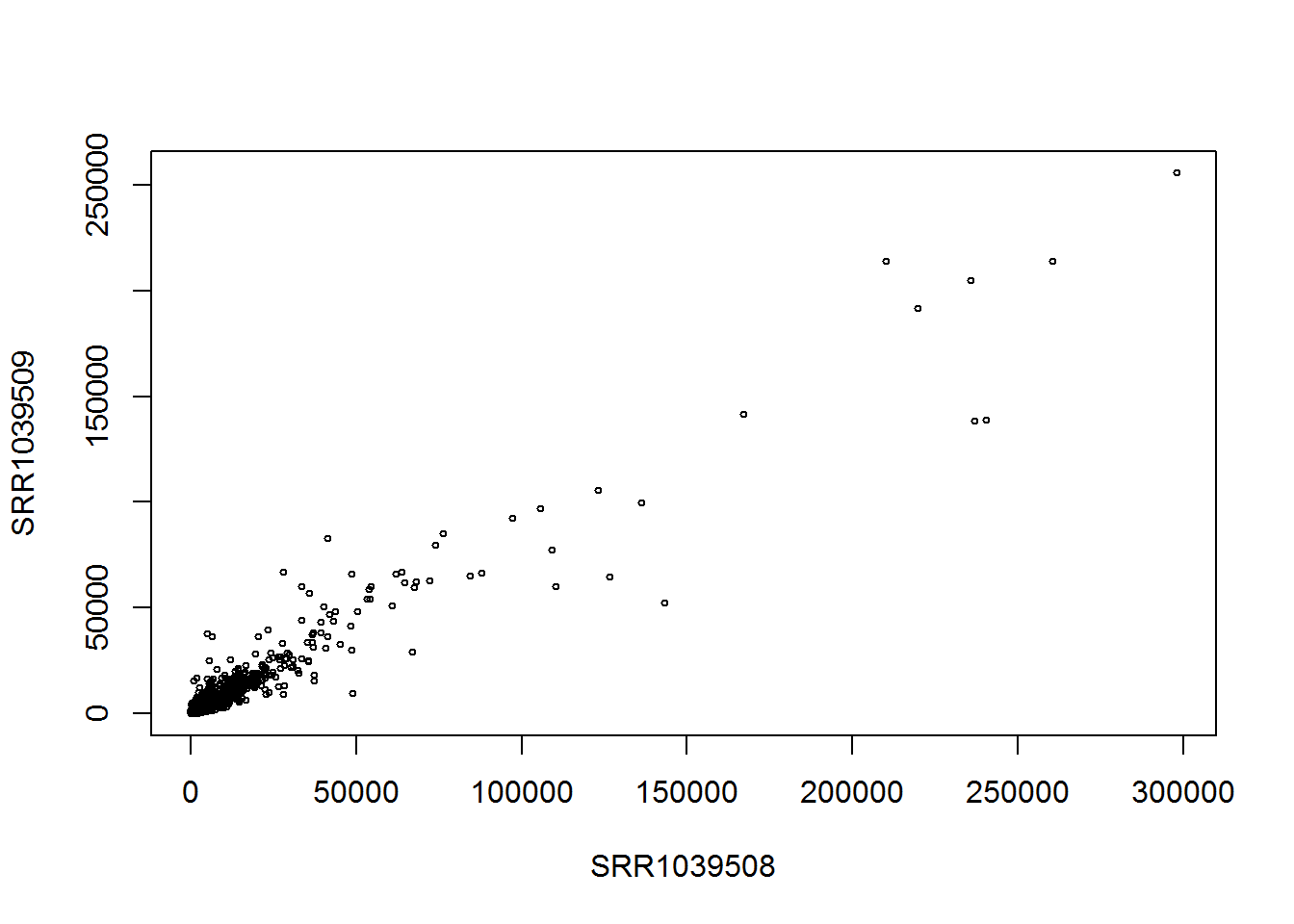Controlling for sequencing depth with normalization and log-transformation

library(DESeq2)
dds <- DESeqDataSet(airway, design= ~ cell + dex)
• Creates a DESeqDataSet from a SummarizedExperiment
• Object can also be created from count matrix and sample table using DESeqDataSetFromMatrix(matrix, colData = sample.table, design = formula)
• estimateSeizeFactors determines a robust estimate of the difference in sequencing depth between samples

dds <- estimateSizeFactors(dds)
sizeFactors(dds)
## SRR1039508 SRR1039509 SRR1039512 SRR1039513 SRR1039516 SRR1039517
##  1.0236476  0.8961667  1.1794861  0.6700538  1.1776714  1.3990365
## SRR1039520 SRR1039521
##  0.9207787  0.9445141
plot(sizeFactors(dds), colSums(counts(dds))) #Compares robust estimate to read totals per sample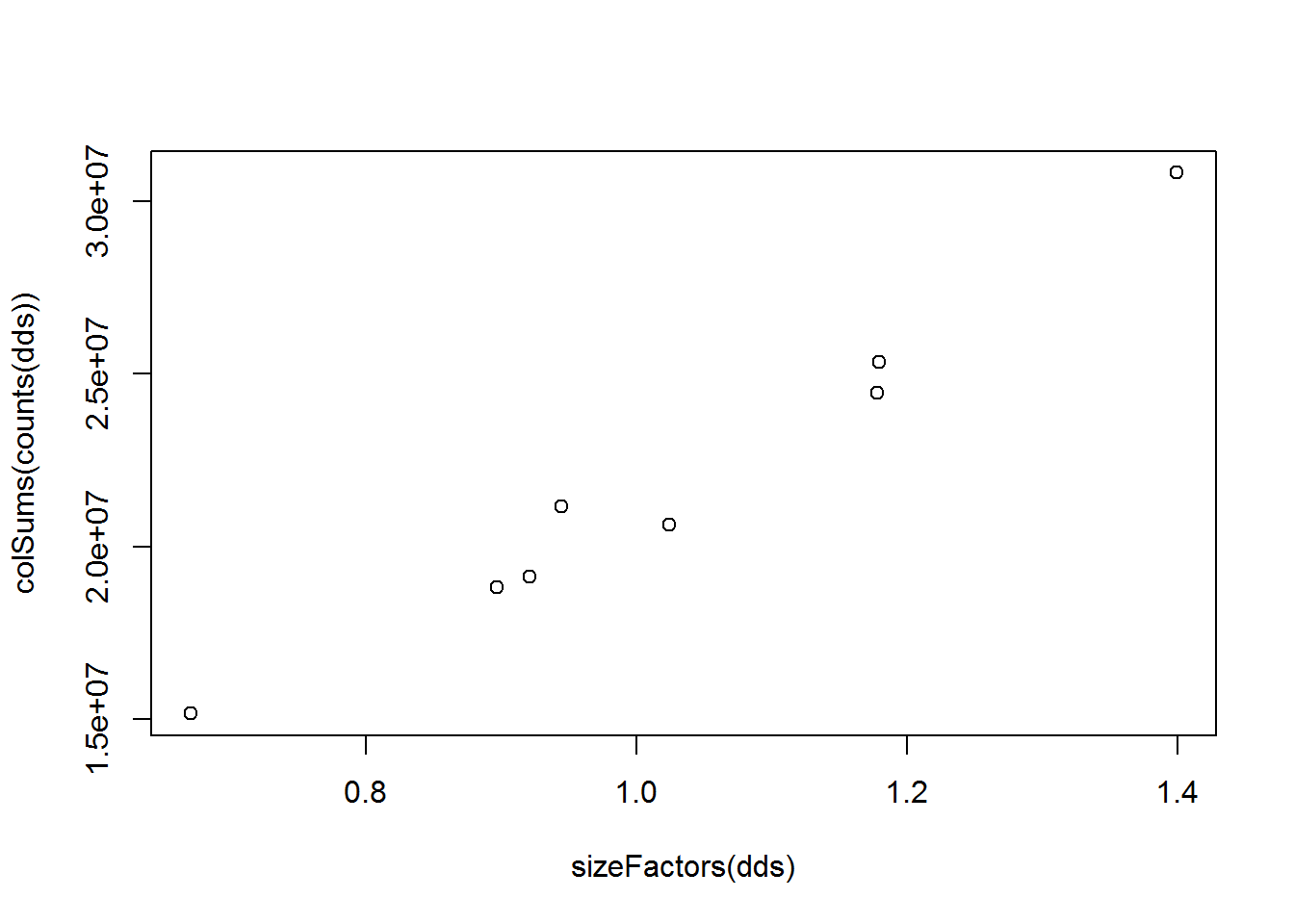• Size factors are calculated using the median, so they are unaffected by large outlier gene counts
• Specifically calculated using the median ratio of a sample to a pseudosample (the geometric mean of each gene across all samples)

exp(median((log(counts(dds)[,1]) - loggeomeans)[is.finite(loggeomeans)])); sizeFactors(dds)
##  1.023648
## SRR1039508
##   1.023648
• log(counts(dds)[,1]) - loggeomeans represents the ratio between counts and the pseudo sample since $$\text{log}(x/y) = \text{log}(x)-\text{log}(y)$$
• exp(median(log(...))) takes the median of this ratio while still log transformed, but converts back with exp
• loggeomeans represnts the pseudosample

loggeomeans <- rowMeans(log(counts(dds))) #The geometric mean is identical to the arithmetic mean of the log transform
hist(log(counts(dds)[,1]) - loggeomeans, #The size factor will be the exp(median) of this distribution
col="grey", main="", xlab="", breaks=40)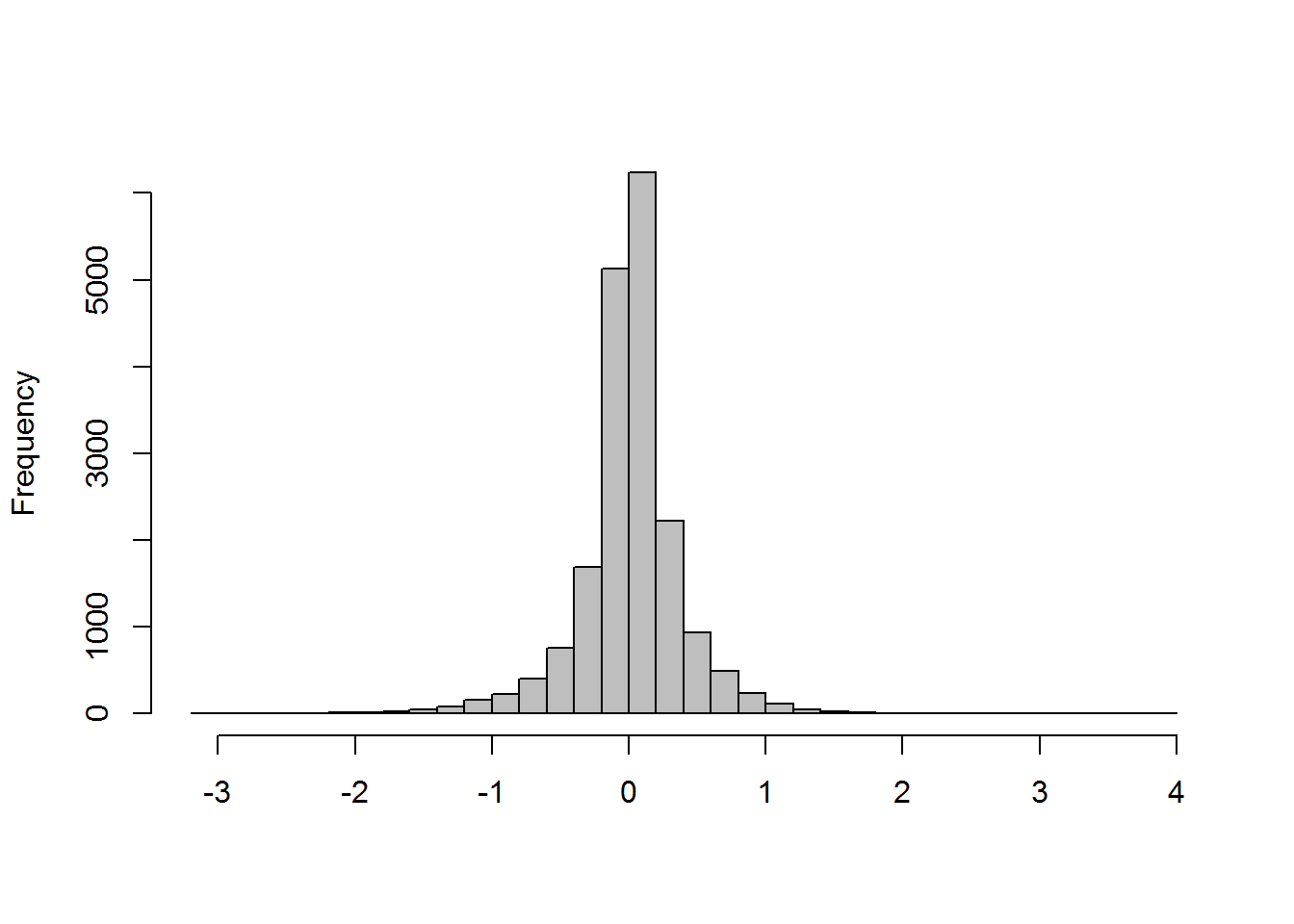#DIGRESSION: The geometric mean is equal to the exponent of the arithmetic mean of the log transformed data
library(psych)
x <- c(1,3,5)
geometric.mean(x); exp(mean(log(x)))
##  2.466212
##  2.466212
• [is.finite(loggeomeans)] removes the genes with any zero counts across samples, since geometric mean will be Inf
• Data can be normalized to sizeEstimates + Divides data point in a column by it’s corresponding size factor

#normalized = T specifies normalization
counts(dds, normalized=TRUE)[1,1]; counts(dds)[1,1]/sizeFactors(dds)
##  663.3142
## SRR1039508
##   663.3142
• Taking the log of the normalized counts may reduce the dominance of high count genes

log.norm.counts <- log2(counts(dds, normalized=TRUE) + 1)
#Alternatively, using DESeq2 function
log.norm <- normTransform(dds)
library(rafalib)
mypar(1,2)
rs <- rowSums(counts(dds))
boxplot(log2(counts(dds)[rs > 0,] + 1)) # not normalized
#+1 represents a pseudocount to avoid problem of log2(0)
boxplot(log.norm.counts[rs > 0,]) # normalized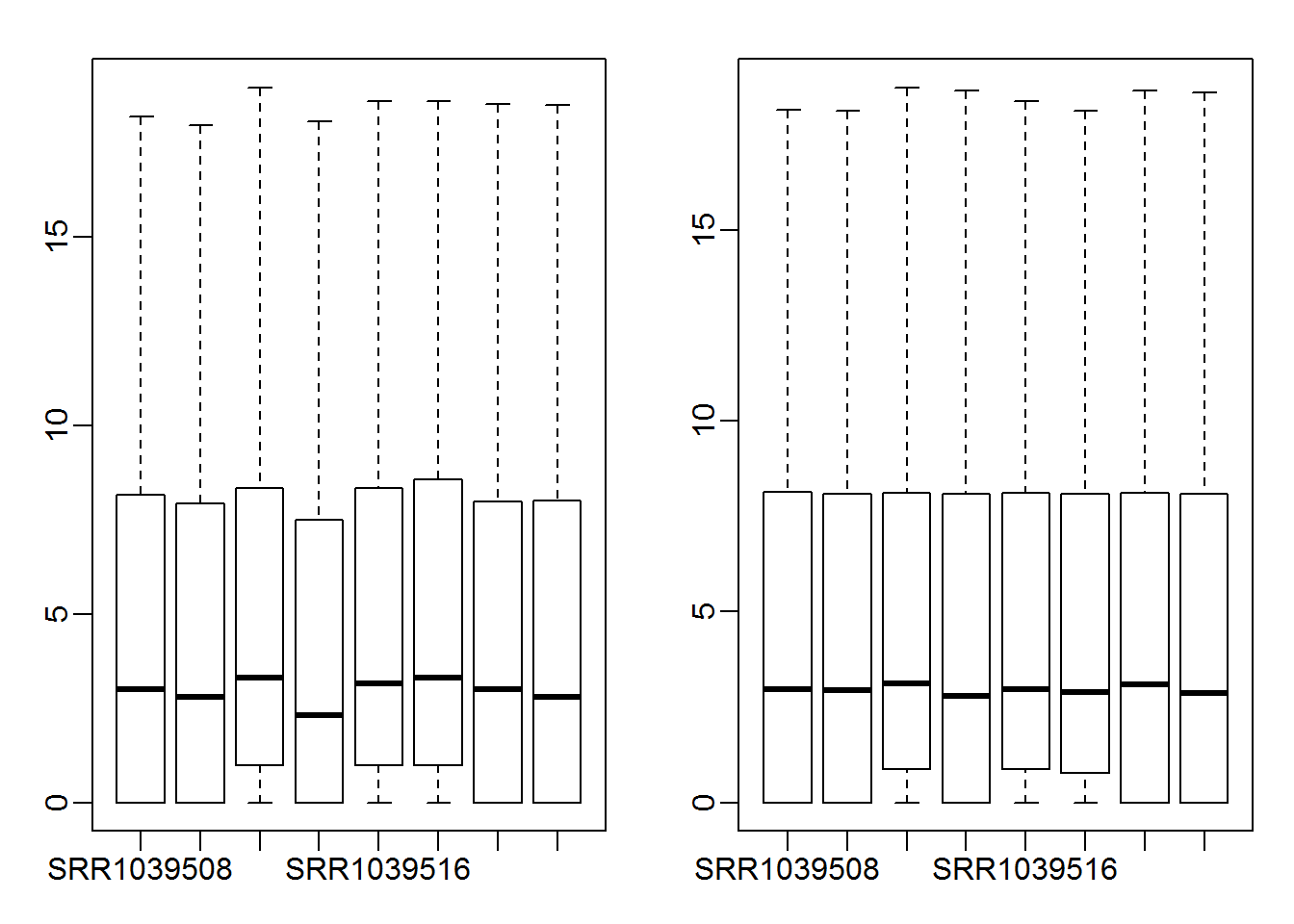library(rafalib)
mypar(1,2)
plot(assay(airway)[,1:2], cex = 0.5)
plot(log.norm.counts[,1:2], cex=0.5)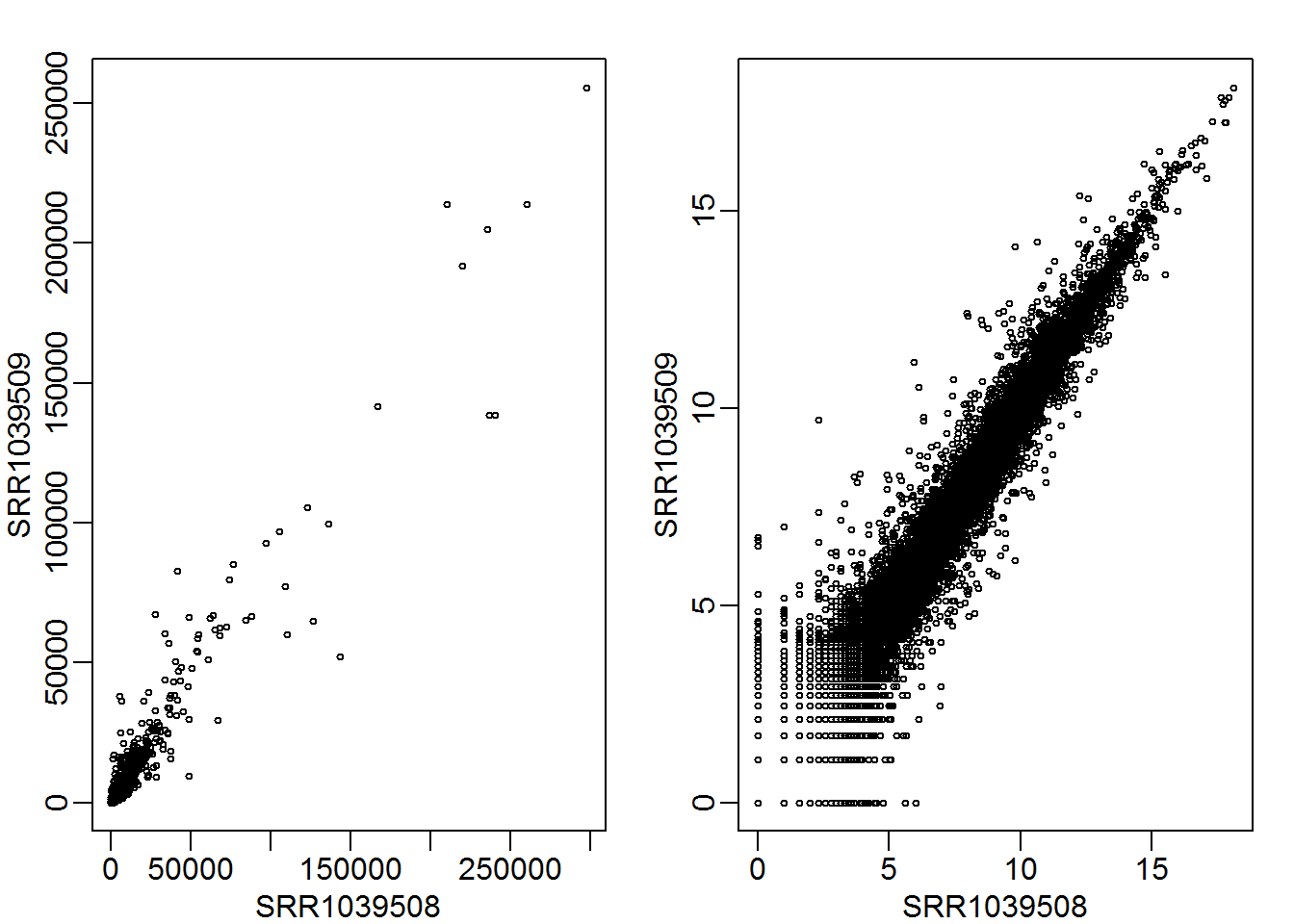• However, notice there is still a disproportionate spread at the lower count values

Transformation with variance stabilization (VST)

• The prinicple feature of variance stabilization is to shrink together the low count data from log-transformed counts
• DESeq2 has two methods for variance stabilization:
• rlog - regularized log, perhaps the best choice when size factors vary greatly
• varianceStabilizingTransformation - faster, particularly for data with many (100s) of samples
rld <- rlog(dds, blind=FALSE)
vsd <- varianceStabilizingTransformation(dds, blind=FALSE)
library(rafalib)
mypar(1,4)
plot(assay(airway)[,1:2], cex = 0.1)
plot(assay(log.norm)[,1:2], cex = 0.1)
plot(assay(rld)[,1:2], cex=.1)
plot(assay(vsd)[,1:2], cex=.1)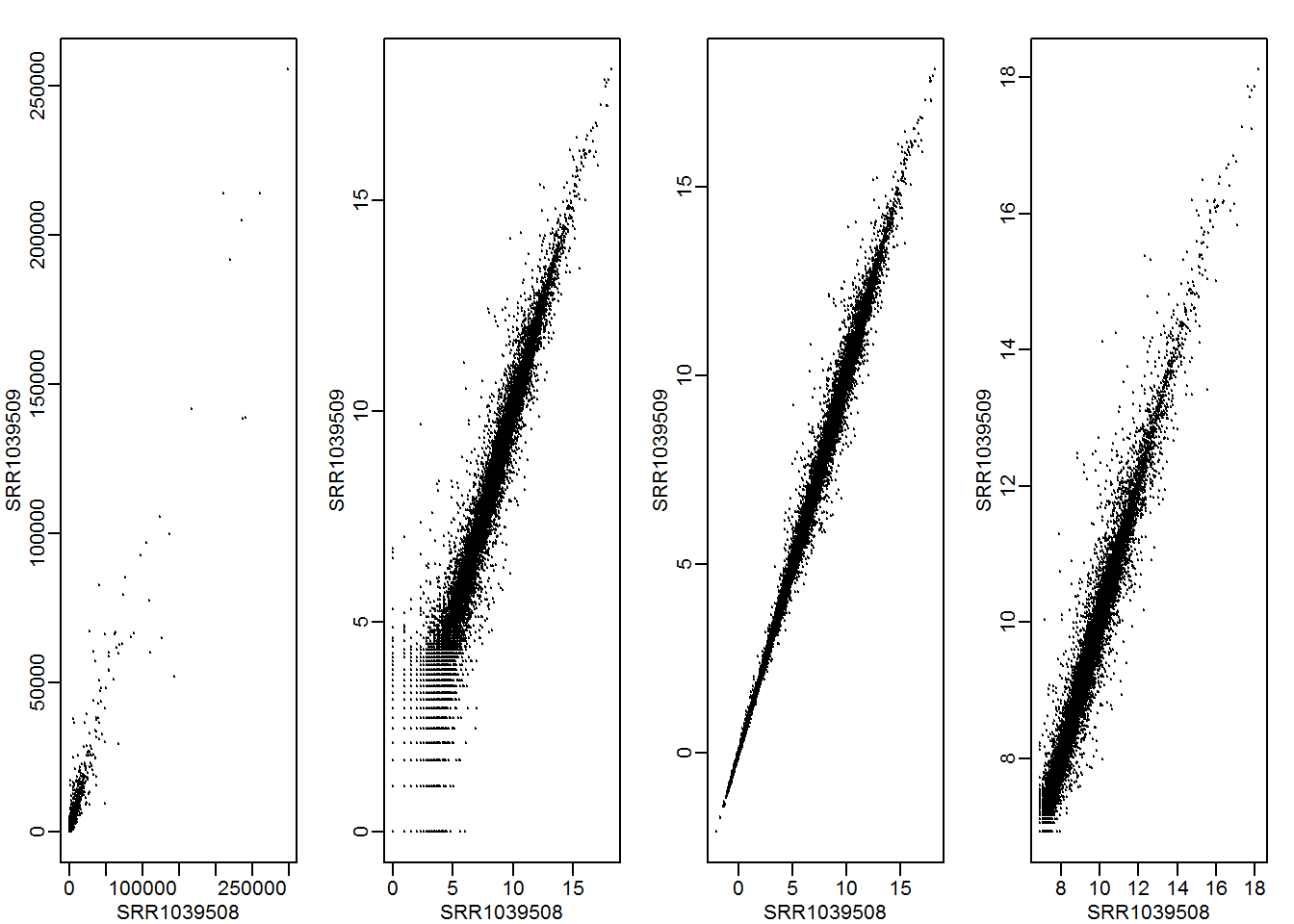• A plot of mean vs. standard deviation for each gene can help show how successful the transformations are

library(gridExtra)
library(vsn)
library(ggplot2)
#Normally, to generate plot, just call meanSdPlot(data, ranks = T)
raw.plot <- meanSdPlot(assay(airway), ranks=FALSE, plot = FALSE)$gg + theme(legend.position="none") + ggtitle("raw") log.plot <- meanSdPlot(assay(log.norm), ranks=FALSE, plot = FALSE)$gg + theme(legend.position="none") + ggtitle("log")
rld.plot <- meanSdPlot(assay(rld), ranks=FALSE, plot = FALSE)$gg + theme(legend.position="none") + ggtitle("rlog") vsd.plot <- meanSdPlot(assay(vsd), ranks=FALSE, plot = FALSE)$gg + theme(legend.position="none") + ggtitle("vsd")
grid.arrange(raw.plot, log.plot, rld.plot, vsd.plot, ncol=4)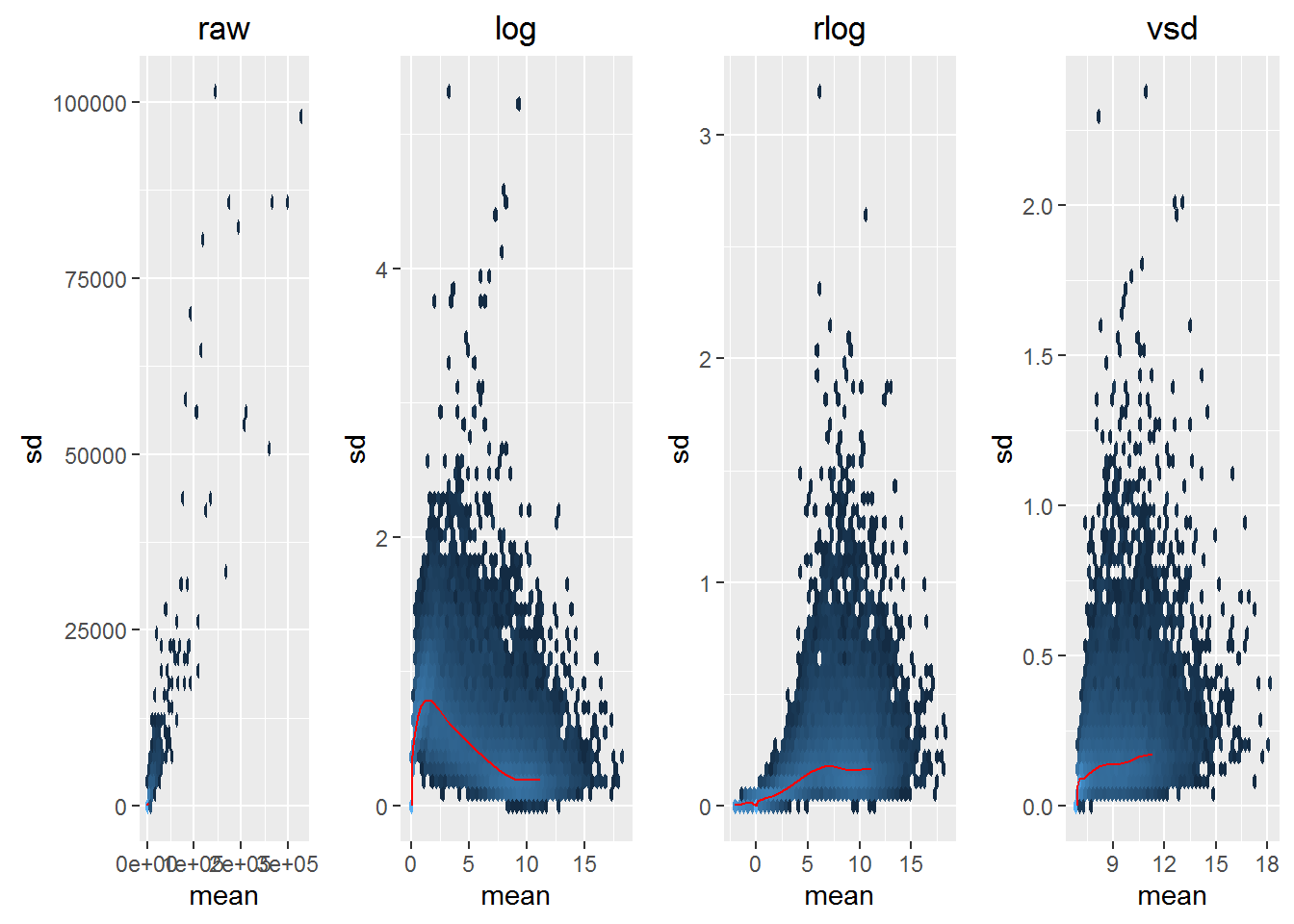• In the raw counts, standard deviation continually increases with mean count number
• The log, rlog, and vsd transforms all stabilize the standard deviation across mean count number
• However, the rlog appears to have the most stable variance across all count numbers

### Exploratory analysis

Correlation analysis

rmeans <- rowMeans(assay(rld)) # row mean of rlog-transformed data
mat <- assay(rld)[rmeans > 1,] # select genes with average count > 1
colnames(mat) <- rld$dex # name the columns of matrix by treatment #Function to sample 1000 points from each sample of a two sample comparison panel.sub <- function(x,y,...) points(cbind(x,y)[sample(length(x),1000),],...) #Function to display R^2 for a two sample comparison panel.cor <- function(x, y, digits = 2, prefix = "", ...) { usr <- par("usr"); on.exit(par(usr)) par(usr = c(0, 1, 0, 1)) r <- abs(cor(x, y)) txt <- format(r, digits = digits) text(0.5, 0.5, txt) } pairs(mat, cex = 0.5, lower.panel=panel.cor, upper.panel=panel.sub)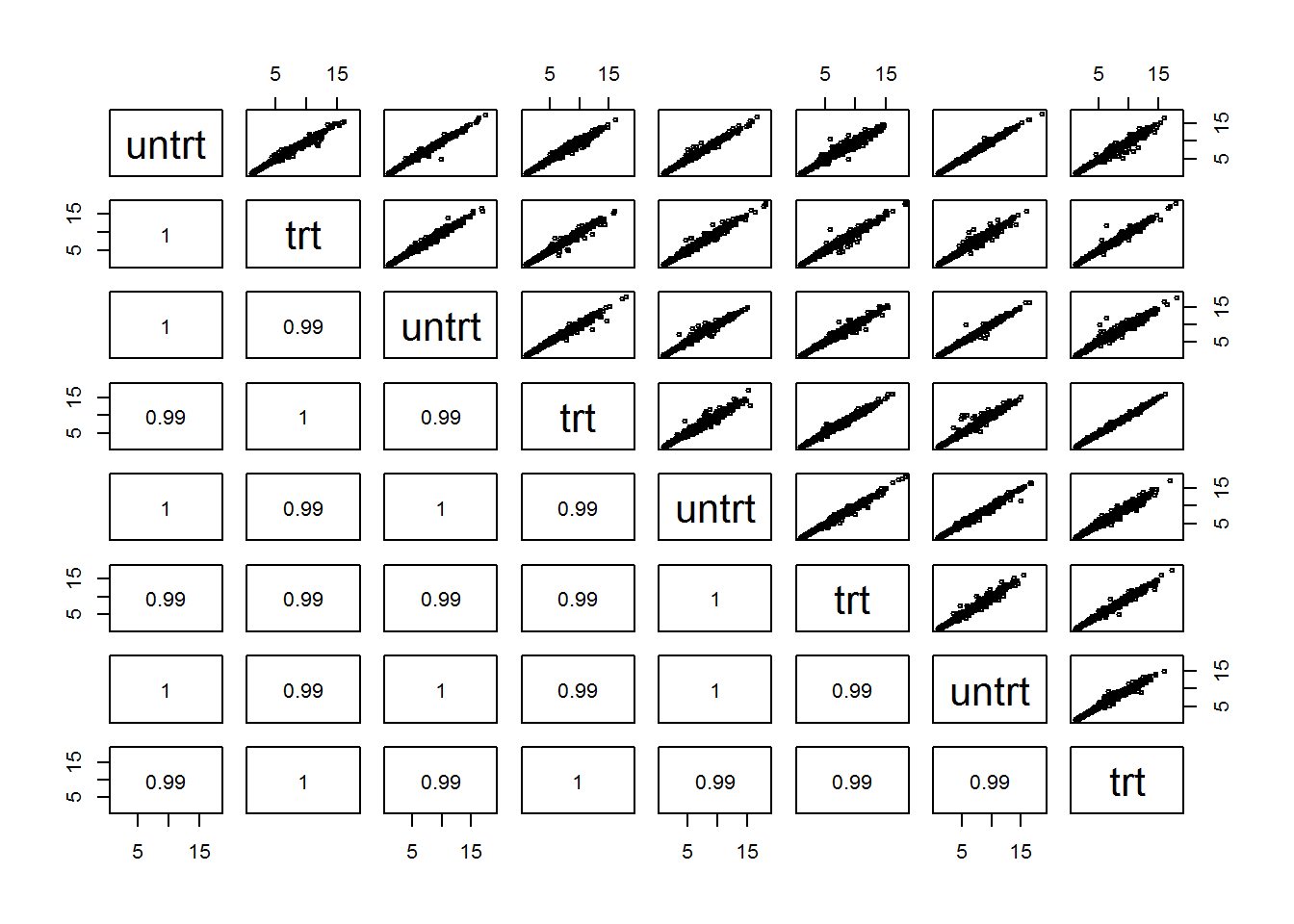Principal component analysis library(ggplot2) data <- plotPCA(rld, intgroup=c("dex","cell"), returnData=TRUE) percentVar <- 100*round(attr(data, "percentVar"),2) makeLab <- function(x,pc) paste0("PC",pc,": ",x,"% variance") ggplot(data, aes(PC1,PC2,col=dex,shape=cell)) + geom_point() + xlab(makeLab(percentVar,1)) + ylab(makeLab(percentVar,2))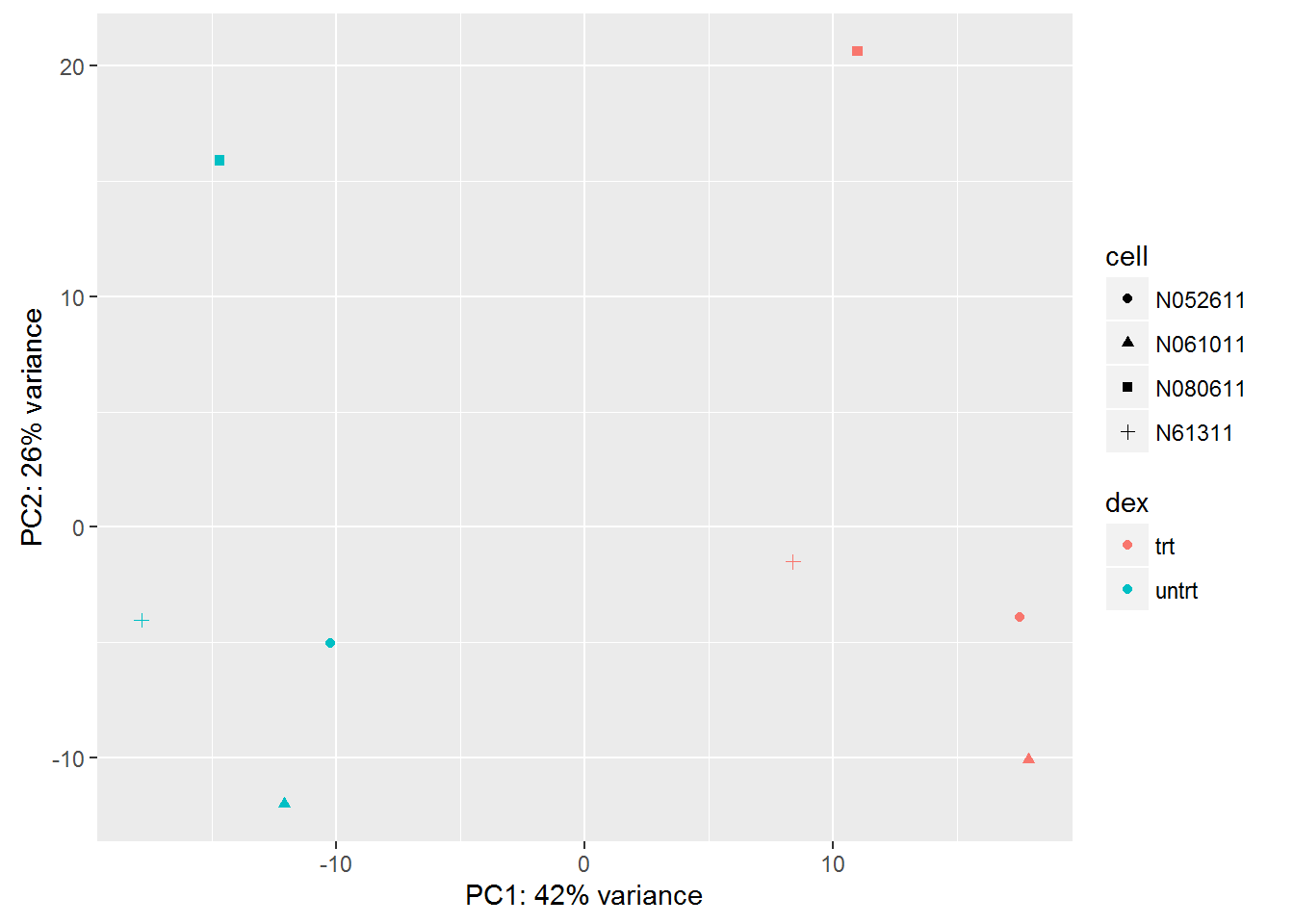Hierarchical clustering library(rafalib) mypar(1,2) plot(hclust(dist(t(assay(log.norm)))), labels=colData(dds)$dex)
plot(hclust(dist(t(assay(rld)))), labels=colData(rld)$dex)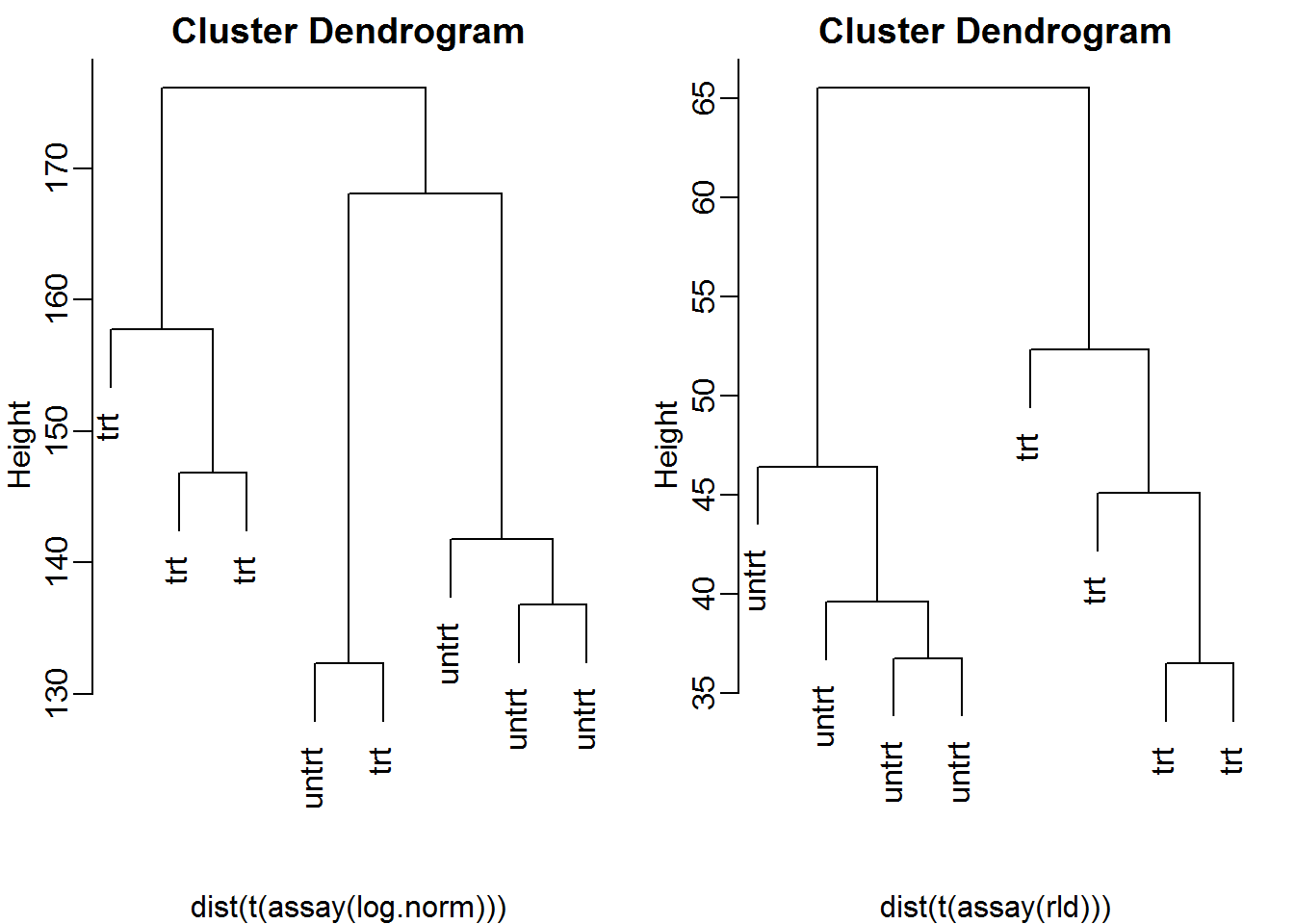Additional example • ReCount database is a site of analysis ready RNA-seq count data • PAPER: Alternative isoform regulation in human tissue transcriptomes. library(Biobase) load(url("https://courses.edx.org/c4x/HarvardX/PH525.5x/asset/wang_eset.RData")) #wang.eset is an ExpressionSet object count.matrix <- exprs(wang.eset)[,10:21] #exprs() extracts matrix of expression values col.data <- pData(wang.eset)[10:21,] #pData() extracts data frame of sample info library(DESeq2) dds <- DESeqDataSetFromMatrix(count.matrix, col.data, design=~cell.type) #Which tissue has the highest size factor? dds = estimateSizeFactors(dds) dds$cell.type[ which.max(sizeFactors(dds)) ]
##  lymph.node
## 8 Levels: cerebellum colon heart liver lymph.node ... skeletal.muscle
#Which tissue clusters with the cerebellum upon VSD transformation
vsd = varianceStabilizingTransformation(dds, blind=FALSE)
plotPCA(vsd, intgroup="cell.type")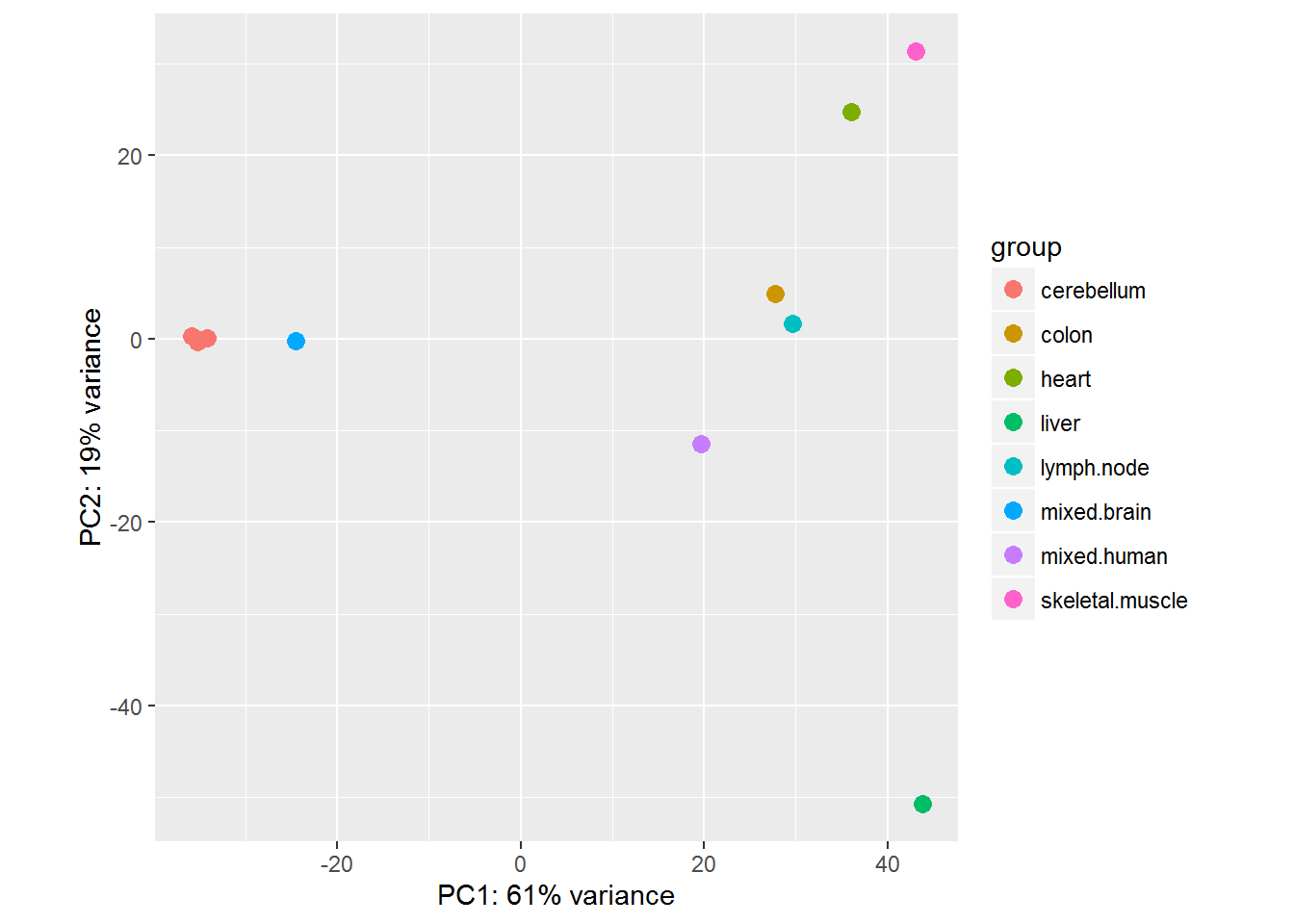rmeans <- rowMeans(assay(vsd))
idx <- c(1,2,10,7,8,9,12) # pick limited set of samples for visualization
mat <- assay(vsd)[rmeans > 1,idx]
colnames(mat) <- vsd\$cell.type[idx] # name the columns of matrix by cell type

pairs(mat, cex = 0.5, lower.panel=panel.cor, upper.panel=panel.sub)